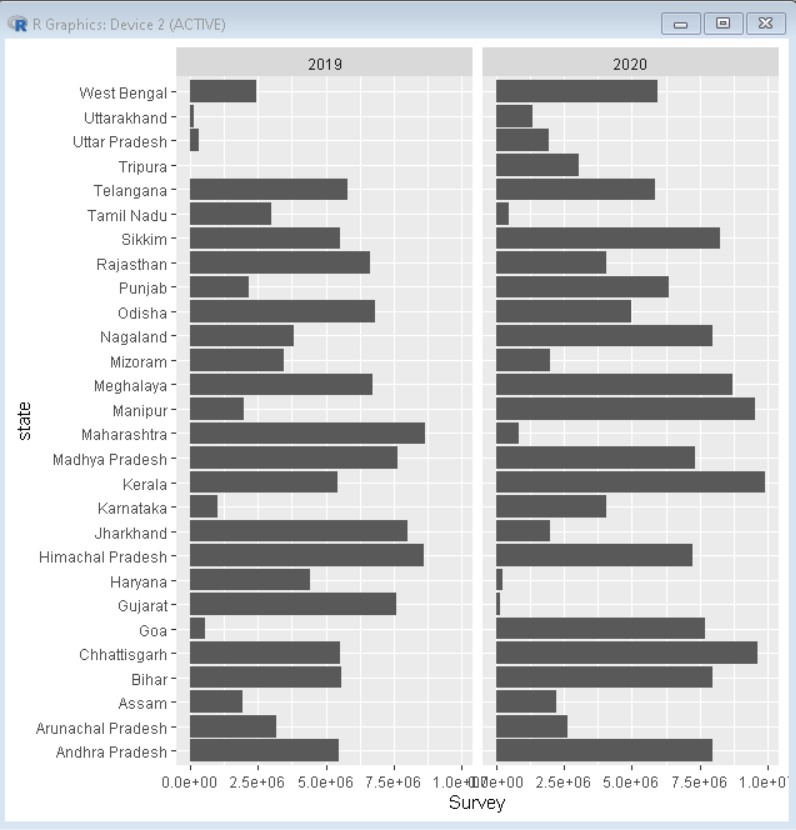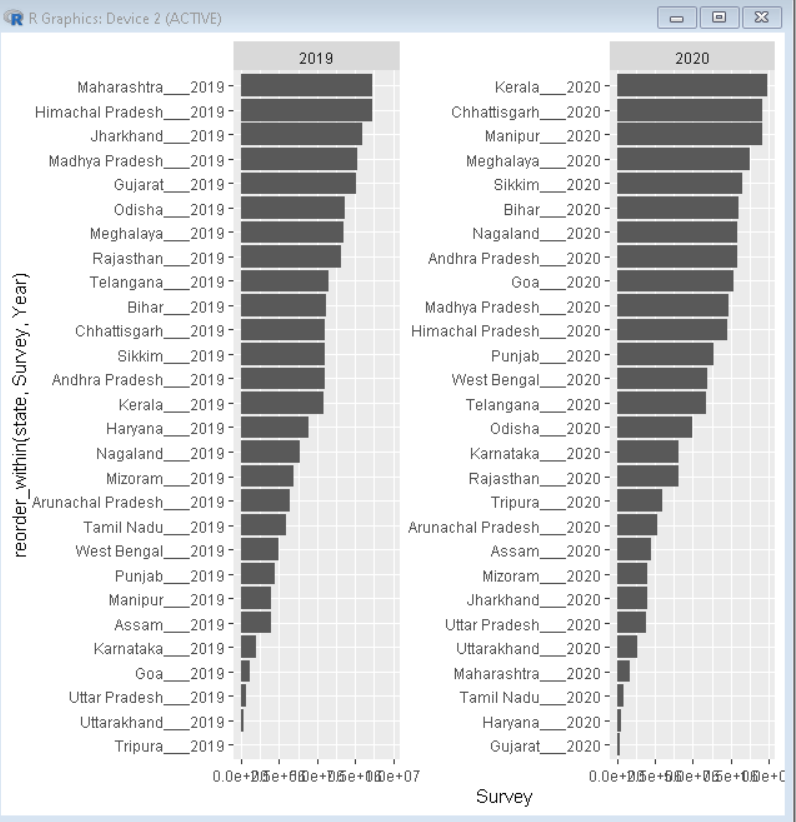Open in App
Not now

# How to reorder barplots with facetting with ggplot2 in R?

• Last Updated : 15 Feb, 2023

In this article, we will discuss how to reorder bar plots with facetting using the ggplot2 package in the R Programming Language. We can draw a facet bar plot by using the geom_col() function with the facet_wrap() function of the ggplot2 package.

Syntax: ggplot( dataframe, aes( x, y ) ) + geom_col() + facet_wrap(~z)

Parameters:

• dataframe: Determines the dataframe to be used for plotting.
• x: Determines the x-axis vector column.
• y: Determines the y-axis vector column.
• z: Determines the variable around which plots have to be divided.

## Creating a basic bar plot

Here, is a basic bar plot with facetting using the facet_wrap() function.

Dataset Used: sample2

## R

 `# Load library tidyverse``library``(tidyverse)` `# create sample data frame``sample_data <- readr::``read_csv``(``'sample2.csv'``)` `# draw a bar plot using geom_col() function``# divide the plot into facts using facet_wrap() function``ggplot``(sample_data, ``aes``(y=state, x=Survey))+``  ``geom_col``()+``  ``facet_wrap``(~Year)`

Output:## Reorder barplot

To reorder the bar plot for better visualization of data, we use the reorder_within() function of the tidytext package of the R Language. The reorder_within() function reorders a column before plotting with faceting, such that the values are ordered within each facet. But this creates a problem that after facetting all the columns that got divided in other facets also co-exist as an empty column for all other facets. To counter that we add scales parameter to facet_wrap() function with its value as free_y or free_x depending on the axis data needs to be freed.

Syntax: ggplot( dataframe, aes( reorder_within(x,y,z) , y ) ) + geom_col() + facet_wrap(~z, scales= “free_y/free_x”)

Parameters:

• dataframe: Determines the dataframe to be used for plotting
• x: Determines the x-axis vector column.
• y: Determines the y-axis vector column.
• z: Determines the variable around which plots have to be divided.

Example:

Here, is a basic bar plot with facetting using the facet_wrap() function. We have also reordered the barplot using the reorder_within() function of the tidytext package.

## R

 `# load library tidyverse and tidytext``library``(tidyverse)``library``(tidytext)` `# create sample data frame``sample_data <- readr::``read_csv``(``'sample2.csv'``)` `# create bar plot with reordering of y-axis variable``# use scales parameter to remove empty variable from y-axis``ggplot``(sample_data, ``aes``(y=``reorder_within``(state,Survey, Year), x=Survey))+``  ``geom_col``()+``  ``facet_wrap``(~Year,scales=``"free_y"``)`

Output:My Personal Notes arrow_drop_up